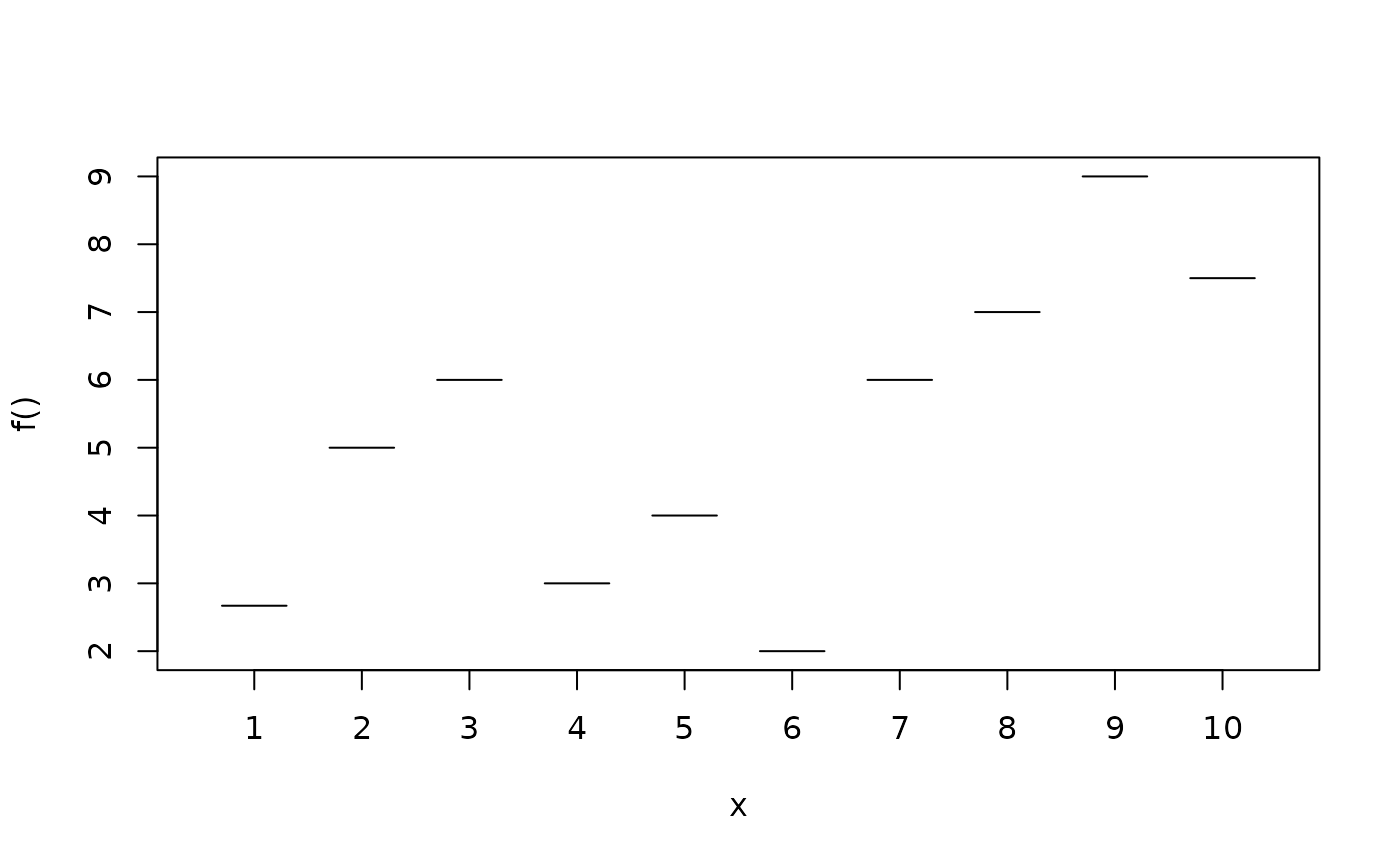Function to plot effects for model terms including factor, or group variables for random effects.

plotblock(x, residuals = FALSE, range = c(0.3, 0.3),
col.residuals = "black", col.lines = "black", c.select = NULL,
fill.select = NULL , col.polygons = NULL, data = NULL,
shift = NULL, trans = NULL, labels = NULL, ...)

## Arguments

x

Either a list of length of the unique factors, where each list element contains the estimated effects for one factor as a matrix, or one data matrix with first column as the group or factor variable. Also formulas are accepted, e.g it is possible to specify the plot with f ~ x or f1 + f2 ~ x. By convention, the covariate for which effects should be plotted, is always in the first column in the resulting data matrix, that is used for plotting, i.e. in the second formula example, the data matrix is cbind(x, f1, f2), also see argument c.select and fill.select.

residuals

If set to TRUE, residuals will be plotted if available. Residuals may be set as an attribute of x named "residuals", where the residuals must be a matrix with first column specifying the covariate, and second column the residuals that should be plotted.

range

Numeric vector, specifying the left and right bound of the block.

col.residuals

The color of the partial residuals.

col.lines

Vector of maximum length of columns of x minus 1, specifying the color of the lines.

c.select

Integer vector of maximum length of columns of x, selects the columns of the resulting data matrix that should be used for plotting. E.g. if x has 5 columns, then c.select = c(1, 2, 5) will select column 1, 2 and 5 for plotting. Note that first element of c.select should always be 1, since this is the column of the covariate the effect is plotted for.

fill.select

Integer vector, select pairwise the columns of the resulting data matrix that should form one polygon with a certain background color specified in argument col. E.g. x has three columns, or is specified with formula f1 + f2 ~ x, then setting fill.select = c(0, 1, 1) will draw a polygon with f1 and f2 as boundaries. If x has five columns or the formula is e.g. f1 + f2 + f3 + f4 ~ x, then setting fill.select = c(0, 1, 1, 2, 2), the pairs f1, f2 and f3, f4 are selected to form two polygons.

col.polygons

Specify the background color for the upper and lower confidence bands, e.g. col = c("green", "red").

data

If x is a formula, a data.frame or list. By default the variables are taken from environment(x): typically the environment from which plotblock is called.

shift

Numeric constant to be added to the smooth before plotting.

trans

Function to be applied to the smooth before plotting, e.g., to transform the plot to the response scale.

labels

Character, labels for the factor levels.

...

Graphical parameters, please see the details.

## Details

Function plotblock draws for every factor or group the effect as a "block" in one graphic, i.e., similar to boxplots, estimated fitted effects, e.g., containing quantiles of MCMC samples, are drawn as one block, where the upper lines represent upper quantiles, the middle line the mean or median, and lower lines lower quantiles, also see the examples. The following graphical parameters may be supplied additionally:

• cex: Specify the size of partial residuals,

• lty: The line type for each column that is plotted, e.g. lty = c(1, 2),

• lwd: The line width for each column that is plotted, e.g. lwd = c(1, 2),

• poly.lty: The line type to be used for the polygons,

• poly.lwd: The line width to be used for the polygons,

• density angle, border: See polygon,

• ...: Other graphical parameters, see function plot.

plot2d, plot3d, plotmap, sliceplot.

## Examples

## Generate some data.
set.seed(111)
n <- 500

## Regressors.
d <- data.frame(fac = factor(rep(1:10, n/10)))

## Response.
d$y <- with(d, 1.5 + rnorm(10, sd = 0.6)[fac] + rnorm(n, sd = 0.6)) if (FALSE) ## Estimate model. b <- bamlss(y ~ s(fac,bs="re"), data = d) summary(b) #> Error in summary(b): object 'b' not found ## Plot random effects. plot(b) #> Error in h(simpleError(msg, call)): error in evaluating the argument 'x' in selecting a method for function 'plot': object 'b' not found ## Extract fitted values. f <- fitted(b, model = "mu", term = "fac") #> Error in fitted(b, model = "mu", term = "fac"): object 'b' not found f <- cbind(d["fac"], f) #> Error in data.frame(..., check.names = FALSE): object 'f' not found ## Now use plotblock. plotblock(f) #> Error in plotblock(f): object 'f' not found ## Variations. plotblock(f, fill.select = c(0, 1, 0, 1), col.poly = "red") #> Error in plotblock(f, fill.select = c(0, 1, 0, 1), col.poly = "red"): object 'f' not found plotblock(f, fill.select = c(0, 1, 0, 1), col.poly = "lightgray", lty = c(2, 1, 2), lwd = c(2, 1, 2)) #> Error in plotblock(f, fill.select = c(0, 1, 0, 1), col.poly = "lightgray", lty = c(2, 1, 2), lwd = c(2, 1, 2)): object 'f' not found ## More examples. plotblock(y ~ fac, data = d, range = c(0.45, 0.45))d <- data.frame(fac = factor(rep(1:10, n/10))) d$y <- with(d, c(2.67, 5, 6, 3, 4, 2, 6, 7, 9, 7.5)[fac])
plotblock(y ~ fac, data = d)plotblock(cbind(y - 0.1, y + 0.1) ~ fac, data = d)